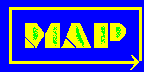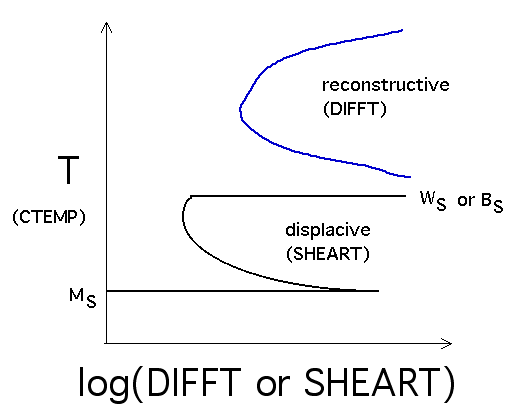# Materials Algorithms Project Program Library

## Program MAP_STEEL_MUCG83

### Provenance of Source Code

Phase Transformations and Complex Properties Group,
Department of Materials Science and Metallurgy,
University of Cambridge,
Cambridge, U.K.

Based on mucg46, and mucg73.

Top | Next

### Purpose

A powerful suite of software for modelling of the thermodynamics and kinetics of solid-state transformations in steels. Calculates Widmanstatten, Bainite and Martensite start temperatures. This program corrects an inconsistency in MUCG73, where a modified free energy function for pure iron was substituted into MUCG46, without making adjustments to other algorithms. Here the program reverts to the free energy function of MUCG46 to restore consistency.

Top | Next | Prev

### Specification

 Language: FORTRAN 77 Product form: Source code and documentation in zipped tape archive file (.tar.gz). or zip file (.zip).

See mucg83.f and included documentation files inside the file mucg83.tar.gz or .zip.

Top | Next | Prev

### Description of Program

MAP_STEEL_MUCG83 is a modified version of MUCG73 and MUCG46.

• The output data contains the concentrations (C, Si, Mn, Ni, Mo, Cr, V, Co, Cu, Al and W) in wt.% and mole fraction (atomic % /100).
• FPRO is the driving force for allotriomorphic ferrite formation by a paraequilibrium mechanism. All driving forces are in units of J/mol and all calculations are for the paraequilibrium state.
• GMAX is the driving force for nucleation (Fig. 6.2b, Ref. 2).
• CTEMP is the temperature in centigrade.
• XNUCLEUS is the optimum nucleus carbon concentration in mole fraction.
• XEQ is the paraequilibrium carbon concentration of austenite in mole fraction.
• XEQ50 is the same as XEQ but allowing for 50 J/mol of strain energy in the ferrite in order to do calculations for Widmanstatten ferrite.
• FTO is the driving force for diffusionless transformation (Fig. 6.2c, Ref. 2).
• XTO is the T-zero carbon concentration in mole fraction (Fig. 1.4, Ref. 2).
• XTO400 is the same as XTO, but with 400 J/mol of stored energy in the ferrite, to allow calculations on bainite (Fig. 1.4, Ref. 2).
• SHEART and DIFFT are the times in seconds of the lower and upper C-curves of the TTT diagram (Ref. 1).

The program asks for compositions (in wt.%) of eleven constituents to be supplied. There are maximum and minimum limits imposed on each constituent as shown :-

Input
Order
Element Max. (wt.%) Min. (wt.%)
1 Carbon 2.0 0.001
2 Silicon 2.5 0.0
3 Manganese 3.5 0.0
4 Nickel 3.5 0.0
5 Molybdenum 1.5 0.0
6 Chromium 3.5 0.0
8 Cobalt 4.0 0.0
9 Copper 4.0 0.0
10 Aluminium 2.0 0.0
11 Tungsten 4.0 0.0

The program will return an error message if these limits are exceeded.

The maximum number of different alloys which can be analysed in a single run is set by the variable J1 (currently 20).

### Plotting a TTT Diagram

These instructions have been compiled by Dr Sangeeta Khare.

The data required to do this include the WS, BS, and MS temperatures, the temperature (CTEMP), the time required to initiate reconstructive transformations (DIFFT) and the time required to initiate displacive transformations (SHEART).

Plotting CTEMP versus SHEART gives the lower C-curve which has an upper cut-off at WS or BS, whichever is greater. Its lower limit is defined by the MS temperature.

The upper C-curve is obtained by plotting CTEMP versus DIFFT

The time axis is usually plotted on a logarithmic scale.Top | Next | Prev

### References

1. H.K.D.H. Bhadeshia, A thermodynamic analysis of isothermal transformation diagrams, Metal Science, 16 (1982) 159-165.
2. H.K.D.H. Bhadeshia, Bainite in Steels, Institute of Materials, London (1992), 1-450.

Top | Next | Prev

### Parameters

#### Input parameters

C - real array of dimension 12
C holds the composition of each alloy:-
C(1) is the Carbon concentration (in weight percent).
C(2) is the Silicon concentration (in weight percent).
C(3) is the Manganese concentration (in weight percent).
C(4) is the Nickel concentration (in weight percent).
C(5) is the Molybdenum concentration (in weight percent).
C(6) is the Chromium concentration (in weight percent).
C(7) is the Vanadium concentration (in weight percent).
C(8) is the Cobalt concentration (in weight percent).
C(9) is the Copper concentration (in weight percent).
C(10) is the Aluminium concentration (in weight percent).
C(11) is the Tungsten concentration (in weight percent).
C(12) is the Ferrite concentration (not entered).

J6 - integer
J6 is the identifying number of each alloy to be examined.

#### Output parameters

None
All output is to the screen.

Top | Next | Prev

### Error Indicators

Allowable composition ranges limited - see Description.

Top | Next | Prev

### Accuracy

No information supplied.

Top | Next | Prev

None.

Top | Next | Prev

### Example

#### 1. Program text

```       N/A - complete program

```

#### 2. Program data

```1
1.0 0 0 0 0 0 0 0 0 0 0

```

#### 3. Program Results

See example.txt for full output.
``` *******  NUMBER    1  *********
C=  1.0000   Si=  0.0000   Mn=  0.0000   Ni=  0.0000   Mo=  0.0000   Cr=  0.0000
V=  0.0000   Co=  0.0000   Cu=  0.0000   Al=  0.0000    W=  0.0000

C=  0.0449   Si=  0.0000   Mn=  0.0000   Ni=  0.0000   Mo=  0.0000   Cr=  0.0000
V=  0.0000   Co=  0.0000   Cu=  0.0000   Al=  0.0000    W=  0.0000

CARBON CONTENT=   0.04485  T10=  0.000000  T20=  0.000000   WGAMMA=  8054.
-------------------------------------------------------------------------------------------------------------------------------
FPRO  FPROA   GMAX  CTEMP  X NUCLEUS  FSON      XEQ    XEQ50   FTO      XTO     VOLF    X44      XTO400       SHEART DIFFT
-2021. -2586. -3105.  200.  0.87E-07  -3073.   0.2018   0.1920 -1171.  0.0903   0.7800 0.603E-01  0.0697  8   0.18E+05   0.25E+24
-1991. -2552. -3065.  205.  0.11E-06  -3038.   0.2005   0.1906 -1146.  0.0914   0.7786 0.605E-01  0.0696  2   0.13E+05   0.65E+23
-1959. -2516. -3025.  210.  0.13E-06  -3001.   0.1991   0.1891 -1121.  0.0915   0.7771 0.601E-01  0.0687  4   0.88E+04   0.18E+23
-1928. -2480. -2987.  215.  0.16E-06  -2964.   0.1978   0.1877 -1096.  0.0906   0.7755 0.596E-01  0.0679  4   0.62E+04   0.49E+22
-1896. -2445. -2959.  220.  0.20E-06  -2927.   0.1965   0.1862 -1071.  0.0893   0.7740 0.590E-01  0.0671  4   0.44E+04   0.14E+22
-1865. -2409. -2913.  225.  0.24E-06  -2890.   0.1951   0.1848 -1046.  0.0880   0.7724 0.585E-01  0.0663  4   0.32E+04   0.41E+21
.
.
.
-14.   -73.  -146.  645.  0.14E-02   -134.   0.0555   0.0204     0.  0.0189   0.1955 0.000E+00  0.0060  3   0.44E+04   0.15E+03
-9.   -56.  -118.  650.  0.14E-02   -105.   0.0533   0.0174     0.  0.0183   0.1612 0.000E+00  0.0050  4   0.12E+05   0.26E+03
-5.   -40.   -89.  655.  0.15E-02    -76.   0.0510   0.0143     0.  0.0175   0.1234 0.000E+00  0.0041  4   0.46E+05   0.58E+03

***** FTO VERSUS TEMPERATURE ****
WIDMANSTATTEN FERRITE START TEMPERATURE  =   345. C
GROWTH LIMITED BAINITE START TEMPERATURE =   356. C
NUCLEATION LIMITED BAINITE START TEMP    =   345. C
MARTENSITE START TEMPERATURE             =   129. C
-------------------------------------------------------------------------------------------------------------------------------
```

Top | Next | Prev

### Auxiliary Routines

MAP_STEEL_GMAAX
MAP_STEEL_HETRO
MAP_STEEL_OMEGA2

#### Utility Subroutines

MAP_UTIL_REED
MAP_UTIL_REEDI

Top | Next | Prev

### Keywords

Widmanstatten, bainite, martensite, start temperature

Top | Next | Prev